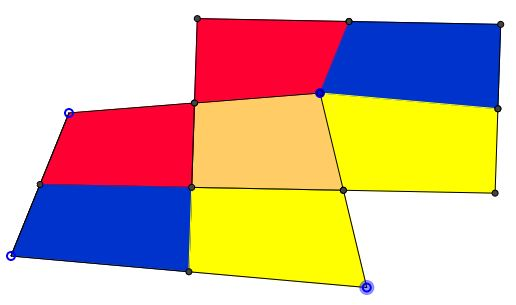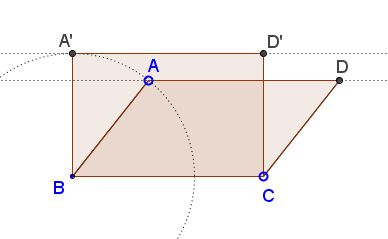### Introduction

Among all quadrilaterals with the same perimeter, square has the largest area.

or, equivalently,

Among all quadrilaterals with the same area, square has the smallest perimeter.

The proof is in three steps:

1. For any quadrilateral (that is not a parallelogram), there is a parallelogram with smaller perimeter but the same area.

2. For every parallelogram (that is not a rectangle), there is a rectangle with larger area but the same perimeter.

3. For every rectangle (that is not a square) there is a square of larger area but the same perimeter.

For any quadrilateral (that is not a parallelogram), there is a parallelogram with smaller perimeter but the same area.### Rectangle among Parallelograms

For every parallelogram, there is a rectangle with larger area but the same perimeter.### Square among Rectangles

For every rectangle (that is not a square) there is a square of larger area but the same perimeter.### Acknowledgment

The above has been communicated to me in private correspondence by Sidney Kung on 1 March, 2014.

• Isoperimetric Theorem and Inequality
• An Isoperimetric theorem
• Isoperimetric theorem and its variants
• Isoperimetric Property of Equilateral Triangles
• Isoperimetric Property of Equilateral Triangles II
• Maximum Area Property of Equilateral Triangles
•